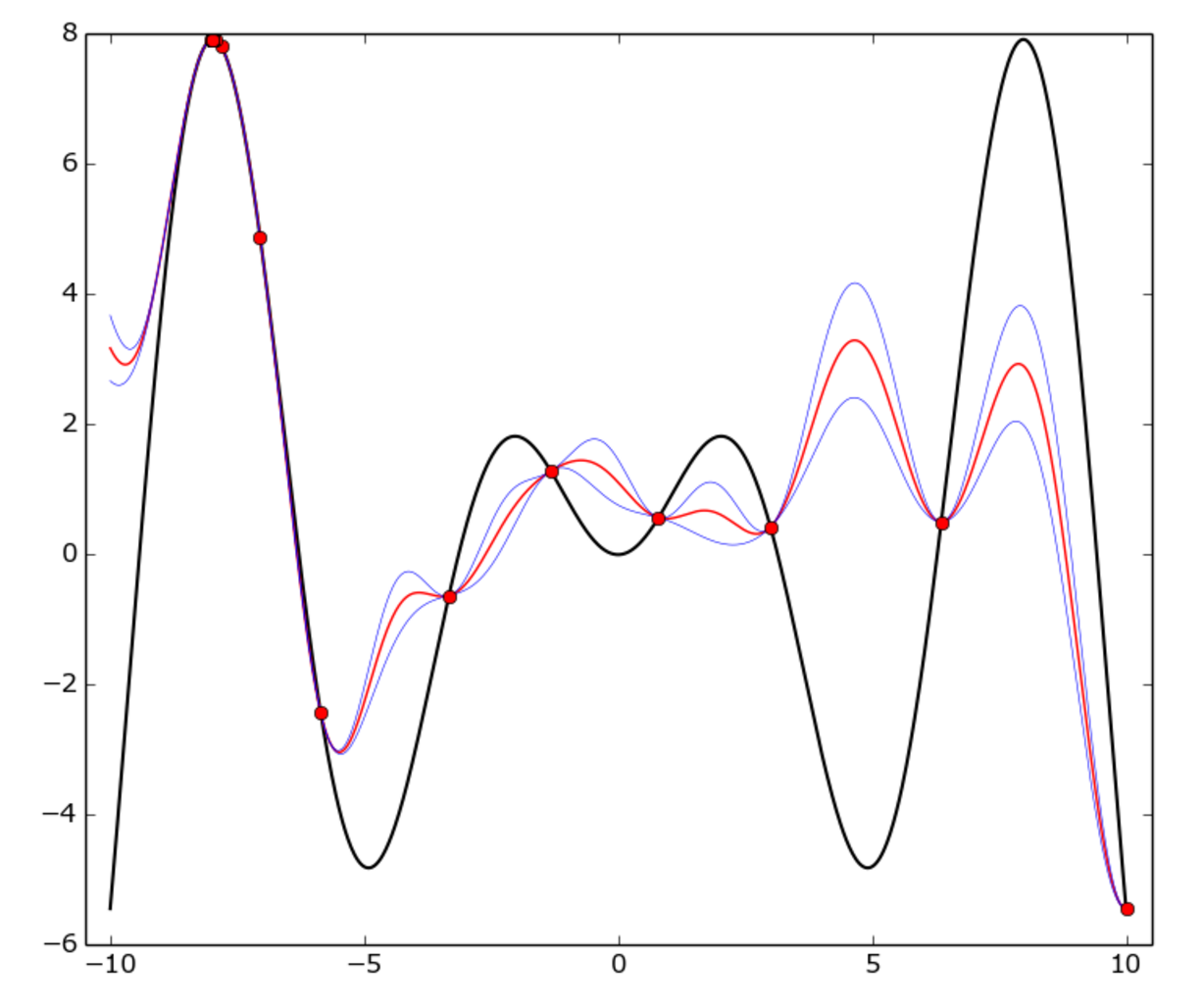2 views

I have constructed a CLDNN (Convolutional, LSTM, Deep Neural Network) structure for the raw signal classification task.

Each training epoch runs for about 90 seconds and the hyperparameters seem to be very difficult to optimize.

I have been researching various ways to optimize the hyperparameters (e.g. random or grid search) and found out about Bayesian Optimization.

Although I am still not fully understanding the optimization algorithm, I feel like it will help me greatly.

I would like to ask a few questions regarding the optimization task.

How do I set up the Bayesian Optimization with regards to a deep network? (What is the cost function we are trying to optimize?)

What is the function I am trying to optimize? Is it the cost of the validation set after N epochs?

Is spearmint a good starting point for this task? Any other suggestions for this task?

I would greatly appreciate any insights into this problem.

by (33.1k points)

Hyperparameter optimization

It is the process of searching for a set of optimal hyperparameters for a learning algorithm. A hyperparameter is a parameter in machine learning algorithms, whose value is used to control the learning process. Our task in deep learning is to find the best value for tuning of hyperparameter.

In your problem, you want to use Bayesian Optimization for hyperparameter tuning. The Bayesian Optimization technique aims to deal with the exploration-exploitation trade-off in the multi-armed bandit problem. In this particular problem, there is an unknown function, which we can evaluate at any point, but each evaluation costs a direct penalty or opportunity cost, and our goal is to find the best hyperparameter in minimum iterations.

Bayesian Optimization is used to build a model of the target function using a Gaussian Process and at each step, it chooses the most "optimal" point based on their GP model.There is a true function in Bayesian optimization that is f(x) = x * sin(x) on [-10, 10] interval. Red dots represent one epoch, the red curve is the GP mean, the blue curve is the mean plus or minus one standard deviation. In this function, the GP model doesn't match with the true function everywhere, but the optimizer fairly quickly identified the "hot" area around -8 and started to exploit it.